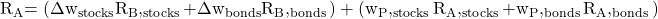Essential Concept 98: Decomposition of Value Added | IFT World
101 Concepts for the Level I Exam

# Essential Concept 98: Decomposition of Value AddedA common way of decomposing value added is between value added due to asset allocation and value added due to security selection.

Let us consider a portfolio of stocks and bonds where the weights allocated to stocks and bonds differ from the benchmark and each asset class is actively managed by selecting individual securities. The total value added due to asset allocation and security allocation is given by:The first term is the value added from asset allocation and the second term is the value added from security selection.

Example: The following information is available for a portfolio comprising equities and bonds:

• Fidelity (equity) mutual fund return: 35.3%
• Benchmark return: 32.3%
• PIMCO (bond) return: -1.9%
• Benchmark return: -2.0%
• Strategic asset allocation: 60% in equities and 40% in bonds.
• Investor’s asset allocation in the actively managed funds: 68% in Fidelity and 32% in PIMCO.

Given the above information, compute the total value added due to active asset allocation and security selection.

Portfolio return = 0.68*0.353 + (-1.9)*0.32= 23.4%

Benchmark return = 0.6*0.323 + (-2.0)*0.4 = 18.6%

Value added = 23.4% – 18.6% = 4.8%

Combined value from asset allocation = 0.08(32.3) + (-0.08)(-2.0) = 2.7%

Combined value added from security selection = 0.68 (3.0) +0.32 (0.1) = 2.1%

LIVE WEEKEND CLASSES *For 2024 Exam candidates*
This is default text for notification bar